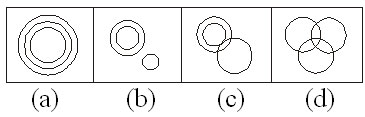# CHSL (10+2) Model Papers Mock Test -5 Reasoning

## CHSL (10+2) Model Papers Mock Test -5 Reasoning

CHSL (10+2) Model Papers Mock Test -5 Reasoning

Directions: Find the related word/letters/number to cornplete the Analogy.

1. Carbon : Diamond :: Corundum : ?

(a) Garnet
(b) Ruby
(c) Pukhraj
(d) Pearl

2. Smoke : Pollution : : War

(a) Victory
(b) Peace
(c) Treaty
(d) Destruction

3. Ink : Pen : : Blood ?

(a) Accident
(b) Doctor
(c) Vein
(d) Donation

4. BOQD : ERTG : : ANPC : ?

(a) DQSF
(b) FSHU
(c) SHFU
(d) DSQF

5. AFKP : ZUPK :: BGLQ : ?

(a) YUQM
(b) XURO
(c) YXWV
(d) YTOJ

6. 182 : ? :: 210 : 380

(a) 342
(b) 272
(c) 240
(d) 156

Directions: Select the related figure from the Answer Figures.

7.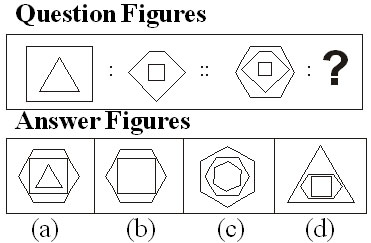8.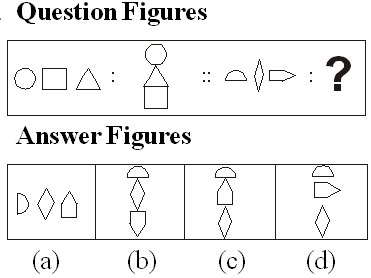9.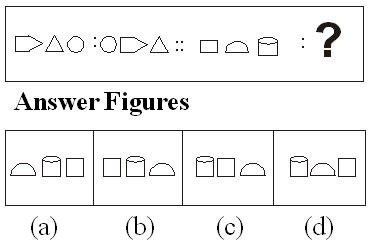10.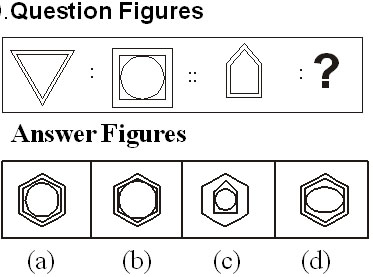11.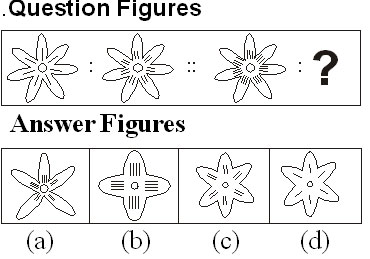Directions: Find the odd one out from the given alterna lives.

12. (a) Mizoram
(b) Sikkim
(c) Kohima
(d) Manipur

13. (a) Amoeba
(b) Bacteria
(c) Germs
(d) Microbes

14. (a) IJCD
(b) WUTS
(c) QRKL
(d) PQMN

15. (a) 8
(b) 42
(c) 49
(d) 35

16. (a) 81 : 243
(b) 16: 64
(c) 64: 192
(d) 25: 75

Directions: Which one of the given responses would be a meaningful order of the following?

17. 1. Book
2. Pulp
3. Timber
4. Jungle
5. Paper

(a) 3, 2, 5, 1, 4
(b) 2, 5, 1, 4, 3
(c) 4, 3, 2, 5, 1
(d) 5, 4, 3, 1, 2

Directions: Arrange the foll lowing words according to dictionary arrangement.

18. 1. Epitaxy
2. Episode
3. Epigene
4. Epitome
5. Epilogue

(a) 1, 2, 3, 4, 5
(b) 3, 5, 2, 1, 4
(c) 5, 4, 2, 1, 3
(d) 3, 2, 5, 4, 1

Directions: A series of figures are given which can be grouped into classes. Select the groups into which the figures can I be class fed.

19.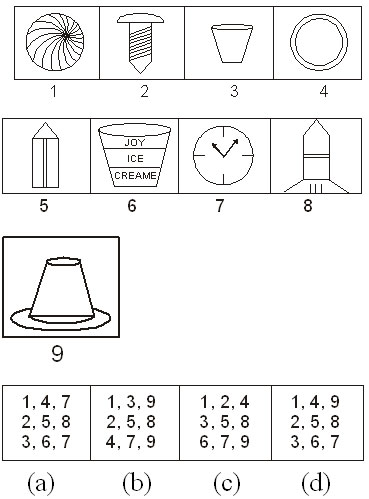20.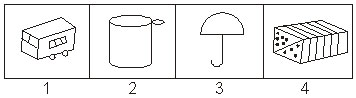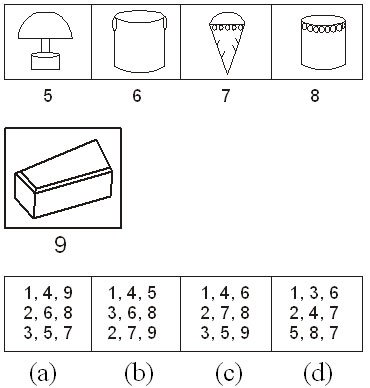21.Directions: Which one set of letters when sequentially placed at the gaps in the given letter series shall complete it?

22. — stt — tt — tts —

(a) tsst
(b) sstt
(c) ttst
(d) tsts

23. Number of letters skipped in between adjacent letters goes on increasing successively by one in the series. Identify the set following the above rule.

(a) AEIMQU
(b) EHKNQT
(c) DINSXC
(d) FHKOTZ

Directions: Find the missing number from the given responses.

24.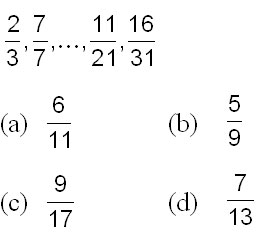25. 25, 50, 30, 45, 35, 40, ?

(a) 30
(b) 35
(c) 40
(d) 45

Directions: Find the missing figure in the series from the given answer figures.

## 26.Question Figures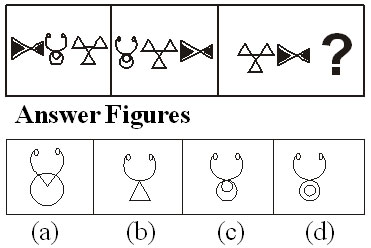27.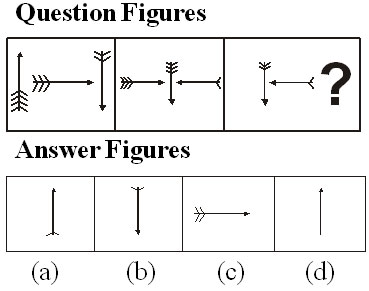Directions: Select the related figure from the answer figures.

28.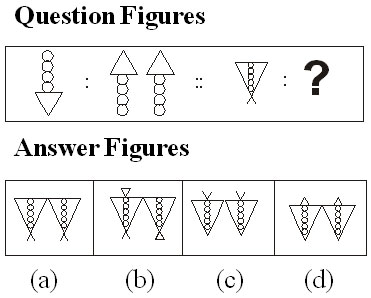29. If ‘+’ stands for division, ‘÷’ stands for multiplication, ‘x’ stands for subtraction and ‘–’ stands for addition, which one of the following is correct?

(a) 18 ÷ 6 – 7 + 5 × 2 = 20
(b) 18 + 6 ÷ 7 × 5 – 2 = 18
(c) 18 × 6 ÷ 7 + 5 – 2 = 16
(d) 18 ÷ 6 × 7 + 5 – 2 = 22

30. If ‘–’ stands for division, ‘+’ for multiplication, ‘÷’ for subtraction and ‘×’ for addition, which one of the following equations is correct?

(a) 18 ÷ 3 × 2 + 8 – 6 = 10
(b) 18 – 3 + 2 × 8 ÷ 6 = 14
(c) 18 – 3 ÷ 2 × 8 + 6 = 17
(d) 18 × 3 + 2 ÷ 8 – 6 = 15

31. If 324 x 150 = 54, 251 x 402 = 48 and 523 x 246 = 120 then 651 x 345 =?

(a) 120
(b) 85
(c) 144
(d) 60

32. Some equations are solved on the basis of certain system. Find out the correct answer for the unsolved equation on that basis.

If 12 × 7 = 408 and 9 × 8 = 207 then 13 x 7 = ?

(a) 190
(b) 91
(c) 901
(d) 109

33. If NOIDA is written as STNIF, how MEERUT can be written in that code?

(a) QIIVYX
(b) RJJWZV
(c) RJJWZY
(d) RIIVYX

34. In a certain code FORGET is written as DPPHCU, how would DOCTOR be written in that code?

(a) BPAUMS
(b) BPAUPS
(c) EMDRPP
(d) BPARPP

35. In a certain code DECEMBER is written as ERMBCEDE, in that code which word will be written as ERMBVENO ?

(a) SEPTEMBER
(b) AUGUST
(c) NOVEMBER
(d) OCTOBER

36. If RED is coded as 6720, then how GREEN would be coded?

(a) 9207716
(b) 1677199
(c) 1677209
(d) 16717209

37. If 9 of the month falls on the day preceding Sunday, on what day will 1st of the month fall?

(a) Friday
(b) Saturday
(c) Sunday
(d) Monday

38. Average marks obtained by 9 students in one row of a class is 45. One student with 35 marks exchanges his seat with a student securing 48 marks. What is the average marks of these 9 students of this row?

(a) 56.4
(b) 46.4
(c) 47.6
(d) 45.6

39. Kamu walks 5 kms straight from her house towards west then turns right and walks 3 kms. There after she takes left turn and walks 2 km. Further, she turns left and walks 3 km. Finally, she turns right and walks 3 kms. In what direction she is now from her house?

(a) West
(b) North
(c) South
(d) East

Directions: In the following question a statement is followed by four inferences. Select the one which is most appropriate.

40. Statement: In the university examination most of the candidates write in Hindi medium. Inferences

(a) All the candidates who appear in this examination write answers in Hindi.
(b) In this examination no candidate writes answers other than in Hindi.
(c) Mostly candidates with Hindi medium appear in this examination.
(d) Some candidates of this examination write in Hindi.

Directions: In the following questions a word is followed by 4 other words, one of which cannot be formed by using the letters of the given word. Find this word.

41. PROSPECTIVE

(a) PEPTIC
(b) PEPPER
(c) VECTOR
(d) RESET

42. APPROXIMATELY

(a) APEX
(b) APPRISE
(c) APPROXIMATE
(d) APRIL

Directions: Which of the answer figures is exactly the mirror image of the given figure when the mirror is held at PQ?

43.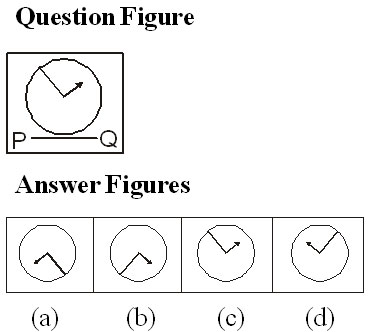44. Amit travelled 15 kms. east­ward, then turned left and travelled 5 kms, then turned left and travelled 15 kms. How far was Amit from the starting point?

(a) 30 kms.
(b) 35 kms.
(c) 15 kms.
(d) 5 kms.

45. At 12.30 the hour hand of a clock faces North and the minute hand faces South. At 2.45 the minute hand will be in which direction?

(a) North-West
(b) West
(c) South-East
(d) East

Directions: Find the missing number from the given responses.

46.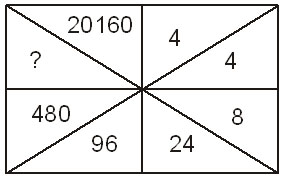(a) 860
(b) 1140
(c) 2880
(d) 3240

47.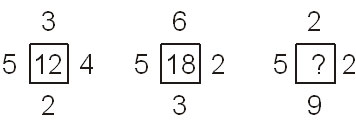(a) 15
(b) 18
(c) 17
(d) 16

48. The trend results are shown at the end of each column. Find out the figure against the missing number.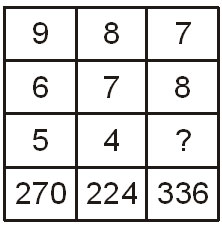(a) 9
(b) 6
(c) 7
(d) 5

49. In the question given below the numbers given at the top follow a certain specific pattern. Study out the pattern and find out the missing number.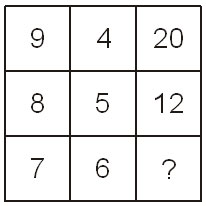(a) 2
(b) 4
(c) 6
(d) 9

50. Which figure represents Rhombus, Quadrilaterals, Polygons?